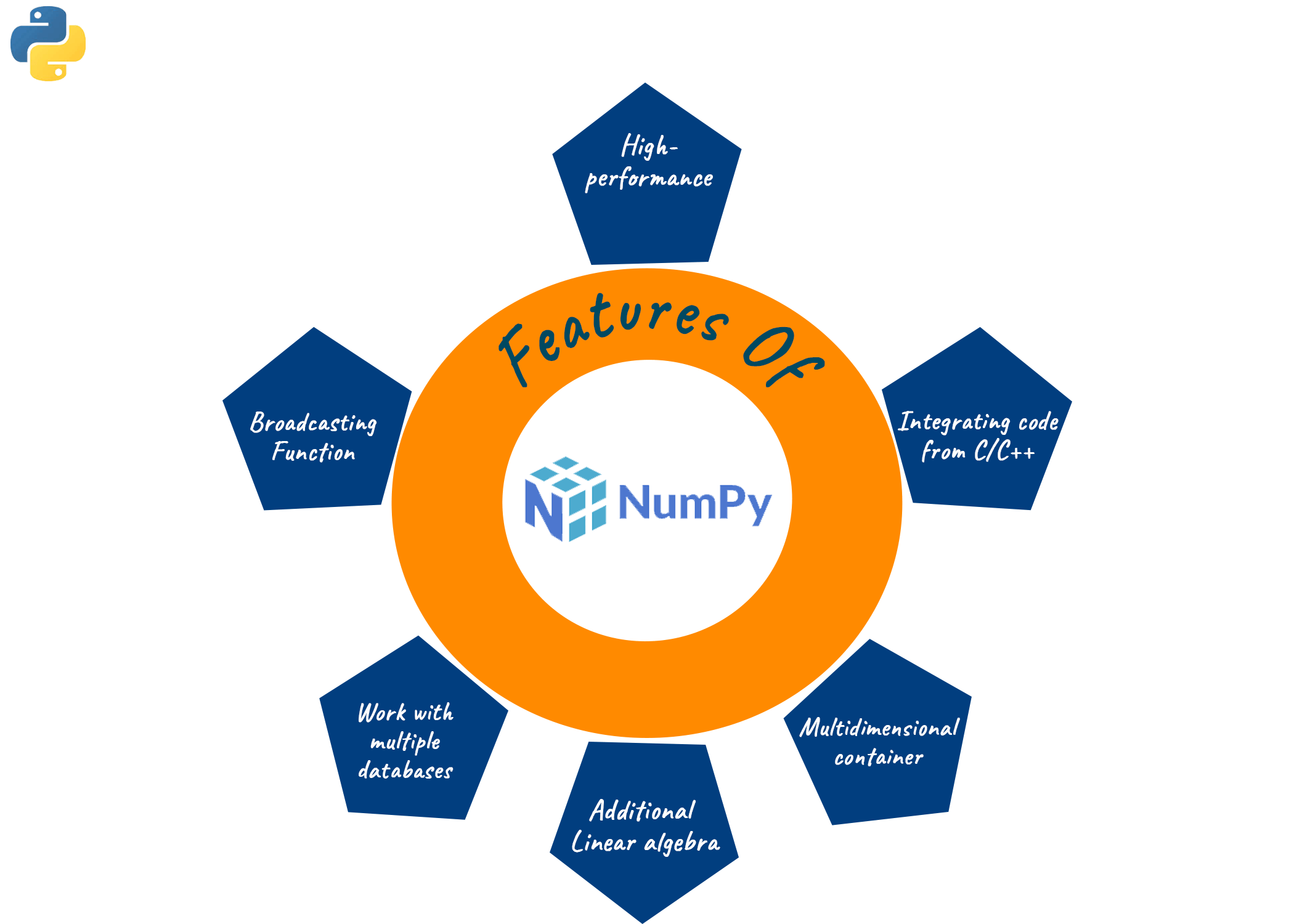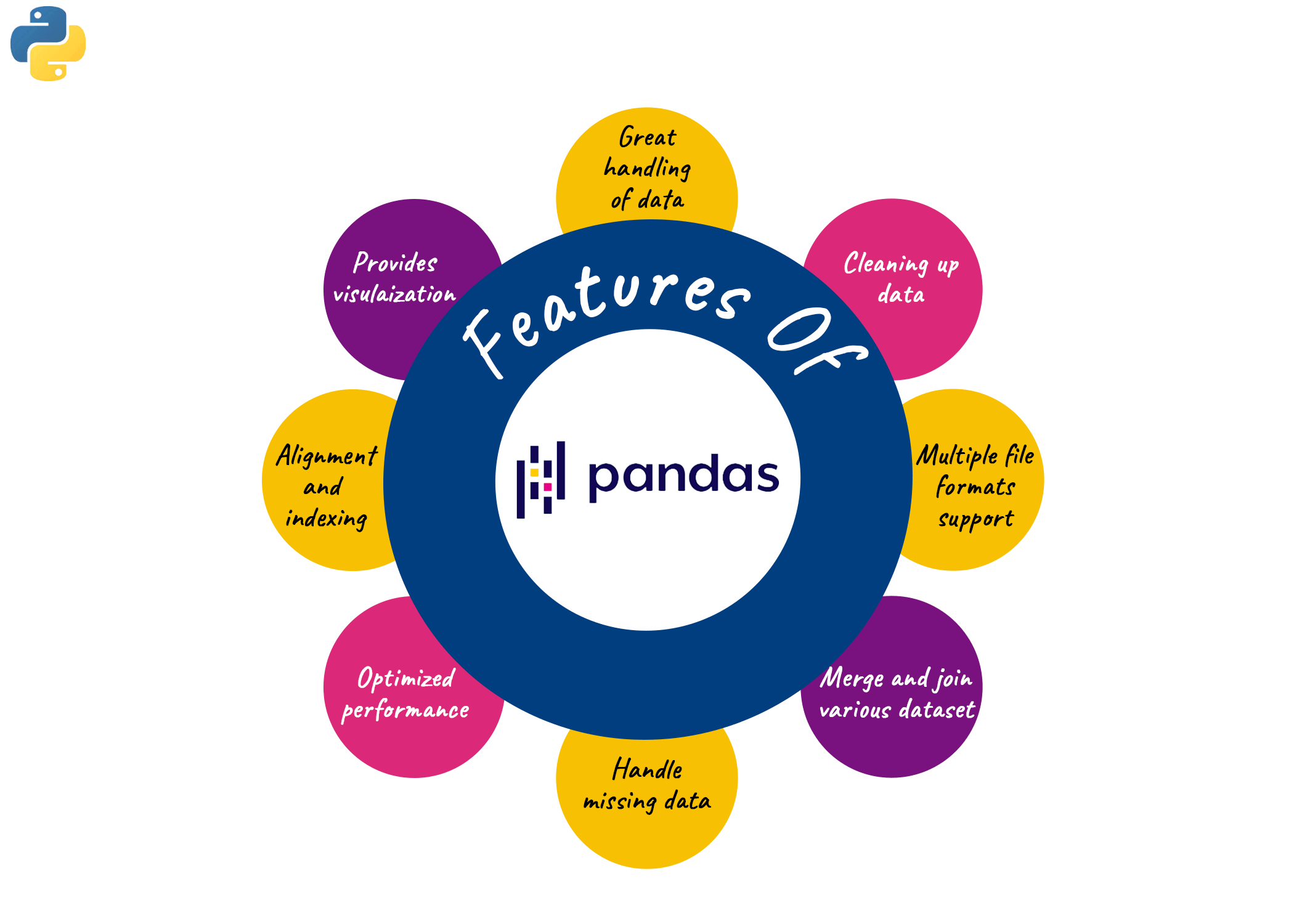# NumPy vs Pandas | 15 Differences Between NumPy and Pandas

Python is indeed the best programming language when it comes to the data science and software development domain. It is because python offers a wide range of benefits such as user-friendly language and easy-to-remember syntax. But apart from this, Python also consists of a huge collection of in-build libraries which enables you to perform the various tasks with minimum effort. NumPy and Pandas are two such popular python libraries. In this article, we will explore the difference between NumPy and Pandas in detail but before that, let us have a brief introduction about them.

## What is NumPy?

NumPy is an abbreviation of Numerical Python. It is one of the most fundamental and powerful python libraries to create and manipulate numerical objects. The basic purpose of designing the NumPy library was to support large multi-dimensional matrices. It helps to perform high-level mathematical functions and complex computations using single and multi-dimensional arrays. NumPy provides innumerable features that reduce the complicated tasks of data analytics, data scientists, researchers, etc.

Below are  some of the common features provided by the NumPy library:

• Enable to work on homogenous datasets using the easy and fast framework
• Helps to build data objects with multiple dimensions
• Provides robust matrix manipulation methods
• Helps to broadcast the applied operations
• Consists of various other packages such as Seaborn, Matplotlib, etc, which can make your work easier and efficient
• Functions as a universal data structure in OpenCV for filter kernels, images, etcNote that NumPy is not part of standard Python installation and therefore you have to install it manually. However, it is quite easy to install and get started with the latest version of NumPy library from the Python repository using PIP as shown below:

```!pip install numpy
```

## What is Pandas?

Pandas is an abbreviation for Python Data Analysis Library. It is an open-source library specially designed for data analysis and data manipulation in Python. Pandas is built on the top of the NumPy package and hence it fundamentally relies on NumPy.

Pandas enable us to read from multiple sources such as Excel, CSV, SQL, and many more. Basically, Pandas possess two types of data objects:

1. Pandas DataFrame: It is a mutable two-dimensional data structure with labeled rows and columns which are generally compared with excel and SQL sheets.
2. Pandas Series: It is a One-dimensional labeled array to store the heterogeneous data elements generally compared with the columns in MS Excel.

Before the inception of Pandas, python used to support very limited data analysis but now, it enables various data operations and manipulates the time series. Basically, Pandas can perform 5 fundamental operations for data analysis: Load, manipulate, prepare, model, and analyze.

Below are some of the common features provided by Pandas library:

• It helps to pivot the datasets
• Pandas enable you to join and merge various datasets
• It enables to handle the missing data and data alignment
• It helps to deal with integrated indexing
• Pandas include the tools for reading and writing data in-memory data structures and multiple file formats
• It supports hierarchical axis indexing for converting high-dimensional data into lower-dimensional dataNote that the individual columns in Pandas are referred to as "Series" and multiple series in the collection are called “DataFrame”. As Pandas are not involved in standard Python installation, you have to externally install it using the PIP utility.

```!pip install pandas
```

## Difference between NumPy and Pandas

 Comparison Parameter NumPy Pandas Powerful Tool A powerful tool of NumPy is Arrays A powerful tool of Pandas is Data frames and a Series Memory Consumption NumPy is memory efficient Pandas consume more memory Data Compatibility Works with numerical data Works with tabular data Performance Better performance when the number of rows is 50K or less Better performance when the number of rows is 500k or more Speed Faster than data frames Relatively slower than arrays Data Object Creates “N” dimensional objects Creates “2D” objects Type of Data Homogenous data type Heterogenous data type Access Methods Using only index position Using index position or index labels Indexing Indexing in NumPy arrays is very fast Indexing in Pandas series is very slow Operations Does not have any additional functions Provides special utilities such as “groupby” to access and manipulate subsets External Data Generally used data created by the user or built-in function Pandas object created by external data such as CSV, Excel, or SQL Industrial Coverage NumPy is mentioned in 62 company stack and 32 developers stack Pandas are mentioned in 73 company stack and 46 developers stack Application NumPy is popular for numerical calculations Pandas is popular for data analysis and visualizations Usage in ML and AI Toolkits can like TensorFlow and scikit can only be fed using NumPy arrays Pandas series cannot be directly fed as input toolkits Core Language NumPy was written in C programming initially Pandas use R language for reference language

### Which is better NumPy or Pandas?

Looking at the above table of differences, it is easily observed that NumPy is more memory efficient in comparison to Pandas. It helps to work on the “N” dimensional data structure which gives it a clear edge over Pandas data frames. When it comes to working in the domain of data science, the NumPy library possesses multiple toolkits such as Tensorflow and Seaborn which can be fed to the models, unlike Pandas. NumPy is also relatively faster than the Pandas series as it takes much time for indexing the data frames.

Pandas have their own importance as the python library, but looking at all the above advantages offered by the NumPy, the conclusion is that NumPy is better than Pandas.

## Conclusion

Python libraries like NumPy and Pandas are often used together for data manipulations and numerical operations. Pandas library is based on NumPy and hence there are significant differences between them. Even though being dependent on each other, we studied various differences between Pandas vs NumPy with their individual features and which is better. For more such amazing articles, do visit Favtutor Blogs.

### FavTutor - 24x7 Live Coding Help from Expert Tutors!